## MATHEMATICS - KCPE 2020 PREDICTION 1 SET 1

1. Write in symbols seven million eight. hundred and fifty thousand and forty?
A. 7085400
B. 7850040
C. 7800540
D. 7008540
2. Write in symbols the numbers which is one million less than 70964205?
A. 6964205
B. 71964205
C. 70864205
D. 69964205
3. What is the place value of digit 9 in 3.73 x 0.4 ?
A. Hundredths
B. Ten thousandths
C. Thousandths
D. Tenths
4. The area of a square plot of land is 2601m2.
What is the perimeter of the square plot?
A. 51m
B. 204m
C. 102m
D. 205m
5. Convert 5/to percentage
A. 50%
B. 62½%
C. 12.5%
D. 6.25%
6. Work out
3.6 ×4.6 ×5.7
0.72 × 0.23 × 0.03
A. 1900
B. 0.19
C. 19 000
D. 190 000
7. Work out √(25×0.32)
A. 1.5
B. 0.15
C. 7.5
D. 0.225
8. Subtract 2½ from 81/3
A. 65/6
B. 61/6
C. 105/6
D. 55/6
9. Work out of 7/8 of 22/7 – (13/163/8)
A. 17/8
B. 213/16
C. 13/16
D. 13/16
10. The price of a dress decreased from sh 240 to sh 200. What was the percentage decrease?
A. 331/3%
B. 162/3%
C. 20%
D. 80%
11. What is the next number in the pattern 1, 5, 17, 53, 161
A. 495
B. 485
C. 493
D. 483
12. The figure below is made up of a quarter circle, a semi-circle and straight lines.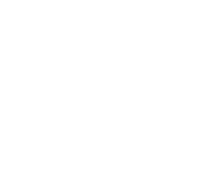What is its perimeter?
A. 97m
B. 86m
C. 75m
D. 56m
13. The diagram below represents Njogu's plot.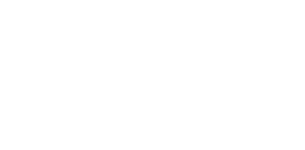He fenced it using 4 strands of barbed wire.
What was the total length of wire used?
A. 80m
B. 304m
C. 240m
D. 320m
14. What is the surface area of the closed cuboid below?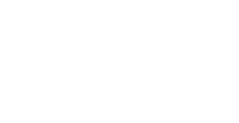A. 118m2
B. 240m2
C. 148m2
D 120m2
15. The radius of a cylinder is 14cm and its volume is 4312cm3. What is its height?
A. 7cm
B. 300 cm
C. 616 cm
D. 49 cm
16. What is the capacity in litres of a container whose shape is a cube of side 20cm?
A. 0.8 litres
B. 8 litres
C. 8000 litres
D. 80 litres
17. Three parcels weigh 867g, 1.362kg and 21/2kg respectively. What is their total mass in kg?
A. 870.862kg
B. 12532kg
C. 37.29kg
D. 4.729kg
18. Johnson bought a goat for sh 1600 and sold it at sh 1760. What was his percentage profit?
A. 90%
B. 160%
C. 10%
D. 110%
19. Cheruiyot paid sh 342 for a watch whose marked price was sh 360. What was the percentage discount?
A. 5%
B. 18%
C. 50%
D. 25%
20. A sales person receives a commission of 5% of the value of his sales. How much commission would he get on sales of sh 75,000?
A Sh. 71 250
B. Sh. 78 750
C. Sh. 15 000
D. Sh. 3750
21. The hire purchase price for a bicycle is sh 5900. Jane paid sh 900 deposit and the balance in 10 equal monthly instalments. How much was each monthly instalment?
A. Sh. 50000
B. Sh. 500
C. Sh. 50
D. Sh. 9000
22. Janice bought the following items 5 kg rice sh 80, 2kg sugar for sh 90, ½kg meat sh 360 and 1kg fat @sh 85. How much did she pay for the items?
A. Sh. 755
B. Sh. 935
C. Sh. 845
D. Sh. 895
23. Kemboi borrowed sh 15000 for a period of 2½years. He was charged simple interest at the rate of 16% per annum. How much interest did he pay?
A. Sh. 9,000
B. Sh. 6,000
C. Sh. 21,000
D. Sh. 600
24. The table below shows distance in kilometres from Nairobi to Kitale.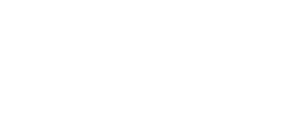What is the distance from Nairobi to Kitale via Kisumu?
A. 336km
B. 85km
C. 501 km
D. 381km
25. Ndereva covered 100 metres in 10 seconds. What was his speed in km/h?
A. 36km/h
B. 10 km/h
C. 360 km/h
D. 100 km/h
26. The temperature of the body is about 63 degrees below the boiling point of water. What is the body temperature?
A. 63°C
B. 37°C
C. 163°C
D. 43°C
27. Work out
b−23b +6 = 4
3            4
A. 212/13
B. 39/13
C. 122/13
D. 212/13
28. Evaluate
4(2x−y)
3y−x
Given that x = 2 y = 3 and z = 4
A. 16/7
B. 4/9
C. 4/7
D. 4/11
29. What is the area of the parallelogram below?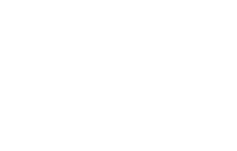A. 20cm2
B. 30 cm2
C. 50 cm2
D. 40 cm2
30. The figure below is a right angled triangle.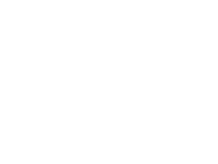Line BC=24cm and the area of the triangle is 120cm2. What is the length of the longest side?
A. 35cm
B. 21cm
C. 35cm
D. 26cm
31. Draw triangle PQR in which PQ = 8cm, QR = 7cm and < PQR =60°. Drop perpendicular from P and let it meet QR at S. measure <SPR.
A. 70°
B. 31o
C. 20o
D. 50o
32. Which statement is not true about the figure below?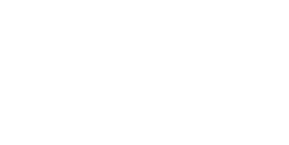A. <a = <h corresponding angles
B. <f = <d -alternate angles
C. <d + <c = 180°
D. <d+ <e = 180°
33. What is the perimeter of the shape alongside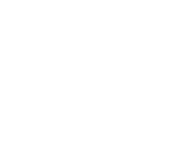A. 177cm
B. 142cm
C. 197cm
D. 122cm
34. The scale used to draw a map is 1 cm represents 60cm, what is this scale in ratio form?
A 1:600
B. 1.60000
C. 1:60
D. 1:6000
35. What is the area of the semicircle below whose diameter is 10 meters? Use π = 3.14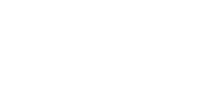A. 39.25m2
B. 29.5m2
C. 31.40m2
D. 15.7m2
36. Find the area of the trapezium below?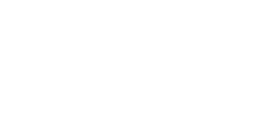A. 750m2
B. 1050m2
C. 525m2
D. 850m2
37. What is the area of the shaded part? Use π = 22/7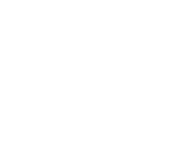A. 154cm2
B. 192.5cm2
C. 346.5cm2
D. 22cm2
38. A trader made a profit of 20% after selling a pair of trouser for sh 240. Calculate his buying price.
A. Sh. 260
B. Sh. 220
C. Sh. 192
D. Sh. 200
39. How many revolutions does a circular ring of radius 14cm make to cover a distance of 1.1km?
Use π = 22/7
A. 2500
B. 6250
C. 250
D. 1250
40. A square garden has an area of 4ha. What will be the length of wire needed to fence round the garden once?
A. 240m
B. 800m
C. 80m
D. 2400m
41. Two sisters inherited sh. 7200 in the ratio 3:5 such that the elder sister received the bigger share. How much money did she get?
A. Sh 2400
B. Sh. 1400
C. Sh. 4500
D. Sh. 2700
42. Two masses of sand are in the ratio 4:5. The smaller mass is 6 tonnes. What is the larger mass?
A. 7.5 tonnes
B. 24 tonnes
C. 30 tonnes
D. 4.8 tonnes
43. A boy's stride is 66cm. how many strides would he make to cover a distance of 131/5m?
A. 5 strides
B. 20 strides
C. 13.2
D. 66cm
44. It takes 4 men 6 hours to dig a ditch. How many hours would it take 8 men to dig the same ditch?
A. 51/3 hours
B. 12 hours
C. 4 hours
D. 3 hours
45. Work to construct a road started on a Monday and ended on a Thursday the same week. The attendance was Monday 29, Tuesday 38. Wednesday 31 and Thursday 38. What was the mean daily attendance?
A. 38
B. 34
C. 136
D. 34.5
46. The pie chart below shows the number of cars, lorries, vans and pick-ups assembled at a Mombasa factory in one month. The factory assembled 50 pick-ups during the month.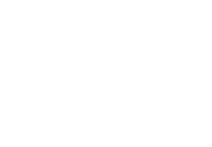How many vehicles altogether were assembled during the month?
A. 360
B. 270
C. 560
D. 240
47. The mean mass of seven boys is 32kg. four weigh 29kg, 31kg, 35kg and 36kg. What is the mean mass of the remaining three boys?
A. 93kg
B. 33kg
C. 31 kg
D. 30kg
48. Arrange 1/2, 7/9, 2/3, 5/6 in an ascending order
A. 5/6, 7/9, 2/3, 1/2
B. 2/31/25/67/9
C. 1/22/37/95/6
D. 1/27/92/35/6
49. In the figure alongside AB is parallel to CD. Angle OPR=40o and angle ORP =20°.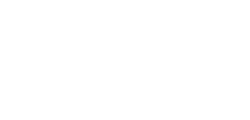What is the measure of angle AOP?
A. 120o
B. 40o
C. 20o
D. 60o
50. Which is the next pattern?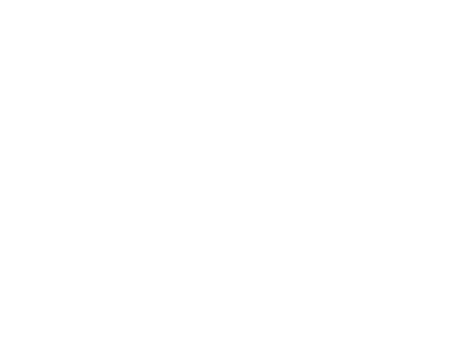## MARKING SCHEME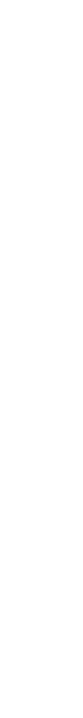• ✔ To read offline at any time.
• ✔ To Print at your convenience
• ✔ Share Easily with Friends / Students